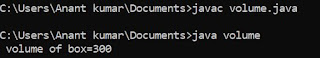## Thursday, 17 May 2018

### Program to print n terms of fibonacci series

/* program to print n terms of fibo series */
# include <stdio.h>
void main ()
{
int a=0,b=1,sum,n ;
int counter=0 ;
clrscr () ;
printf ("\n %d",a) ;
printf ("\n %d",b) ;
printf ("\n how many terms you want to print:") ;
scanf ("%d",& n) ;
while ( counter<n-2 )
{
sum =a+b ;
printf ("\n %d", sum) ;
a=b ;
b=sum ;
counter++ ;
}
getch () ;
}